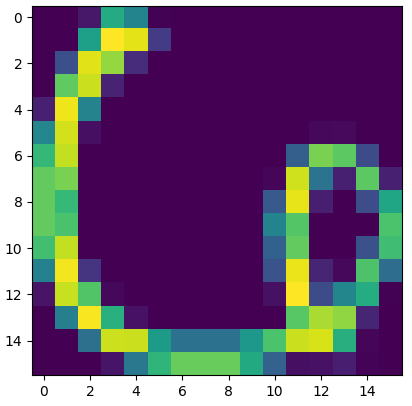Hi, All.

To make experiments more convience, I’ve tried to write a Dataset that can be feeded to dataloader.
However, my dataset can read data correctly while the dataloader distorted the images as follows.
Images in my dataset is 90001616. (gray images)
I want to find out how does dataloader transform the dataset into 9000116*16, but I can’t find the code.

Image in my dataset:Here is my code:

`````` # training set
transform = transforms.Compose([transforms.Scale(28), transforms.ToTensor(), ])
# split data

# kernel code in USPS

# process and save as torch files
print('Processing...')
traindata = torch.from_numpy(data['data'].transpose())

traindata = traindata.view(16,16,-1).permute(2,1,0)
trainlabel = torch.from_numpy(data['labels'])